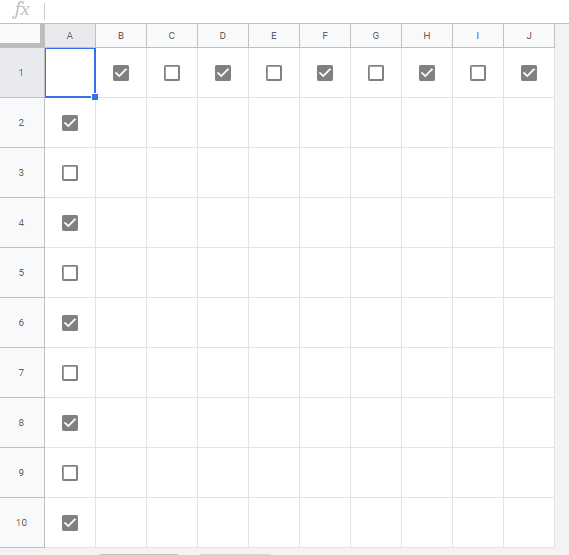Gists

These are the sample scripts for hiding and deleting rows and columns on Google Spreadsheet using Google Apps Script. I sometimes see the questions for hiding and deleting rows and columns on Spreadsheet at Stackoverflow. So here, I would like to introduce the sample scripts for this.

In this case, when the process costs of the scripts created by using Spreadsheet service and Sheets API are compared, the cost of script created by Sheets API is much lower than that of script created by Spreadsheet service. So when the rows and columns of your Spreadsheet is large and you can use Sheets API, I recommend to use Sheets API.

## Sample situation

As the sample situation, the following sample Spreadsheet is used.The 1st row and the column “A” are used as the header row and header column, respectively. Each header has the checkboxes. This sample Spreadsheet is used for the following scripts.

## Hide rows

The rows of checked checkboxes of the column “A” are hidden.

### Sample script 1

In this sample script, hideRow of Spreadsheet service is used.

// Hidden rows using Spreadsheet service.
const checkBoxColumn = 1;
const sheetName = "Sheet1";

for (let r = 2; r <= sheet.getLastRow(); r++) {
const range = sheet.getRange(r, checkBoxColumn);
if (range.isChecked()) sheet.hideRow(range);
}
}


### Sample script 2

In this sample script, updateDimensionProperties of the batchUpdate method in Sheets API is used. Before you use this script, please enable Sheets API at Advanced Google services.

// Hidden rows using Sheets API.
function hideRows_with_SheetsAPI() {
const checkBoxColumn = 1;
const sheetName = "Sheet1";

const sheet = ss.getSheetByName(sheetName);
const sheetId = sheet.getSheetId();
const values = sheet
.getRange(2, checkBoxColumn, sheet.getLastRow() - 1)
.getValues();
const requests = values.reduce((ar, [a], i) => {
if (a === true) {
const offset = i + checkBoxColumn;
ar.push({
updateDimensionProperties: {
range: {
sheetId: sheetId,
startIndex: offset,
endIndex: offset + 1,
dimension: "ROWS",
},
properties: { hiddenByUser: true },
fields: "hiddenByUser",
},
});
}
return ar;
}, []);
}


## Hide columns

The columns of checked checkboxes of the 1st row are hidden.

### Sample script 1

In this sample script, hideColumn of Spreadsheet service is used.

// Hidden columns using Spreadsheet service.
const checkBoxRow = 1;
const sheetName = "Sheet1";

for (let c = 2; c <= sheet.getLastColumn(); c++) {
const range = sheet.getRange(checkBoxRow, c);
if (range.isChecked()) sheet.hideColumn(range);
}
}


### Sample script 2

In this sample script, updateDimensionProperties of the batchUpdate method in Sheets API is used. Before you use this script, please enable Sheets API at Advanced Google services.

// Hidden columns using Sheets API.
function hideColumns_with_SheetsAPI() {
const checkBoxRow = 1;
const sheetName = "Sheet1";

const sheet = ss.getSheetByName(sheetName);
const sheetId = sheet.getSheetId();
const values = sheet
.getRange(checkBoxRow, 2, 1, sheet.getLastColumn() - 1)
.getValues();
const requests = values.reduce((ar, e, i) => {
if (e === true) {
const offset = i + checkBoxRow;
ar.push({
updateDimensionProperties: {
range: {
sheetId: sheetId,
startIndex: offset,
endIndex: offset + 1,
dimension: "COLUMNS",
},
properties: { hiddenByUser: true },
fields: "hiddenByUser",
},
});
}
return ar;
}, []);
}


## Delete rows

The rows of checked checkboxes of the column “A” are deleted. As an important point, when the rows are deleted, the row index is changed. By this, when the rows are deleted from the bottom row, the script becomes simpler.

### Sample script 1

In this sample script, deleteRow of Spreadsheet service is used.

// Delete rows using Spreadsheet service.
const checkBoxColumn = 1;
const sheetName = "Sheet1";

for (let r = sheet.getLastRow(); r >= 2; r--) {
const range = sheet.getRange(r, checkBoxColumn);
if (range.isChecked()) sheet.deleteRow(r);
}
}


### Sample script 2

In this sample script, deleteDimension of the batchUpdate method in Sheets API is used. Before you use this script, please enable Sheets API at Advanced Google services.

// Delete rows using Sheets API.
function deleteRows_with_SheetsAPI() {
const checkBoxColumn = 1;
const sheetName = "Sheet1";

const sheet = ss.getSheetByName(sheetName);
const sheetId = sheet.getSheetId();
const values = sheet
.getRange(2, checkBoxColumn, sheet.getLastRow() - 1)
.getValues();
const requests = values
.reduce((ar, [a], i) => {
if (a === true) {
const offset = i + checkBoxColumn;
ar.push({
deleteDimension: {
range: {
sheetId: sheetId,
startIndex: offset,
endIndex: offset + 1,
dimension: "ROWS",
},
},
});
}
return ar;
}, [])
.reverse();
}


## Delete columns

The columns of checked checkboxes of the 1st row are deleted. As an important point, when the columns are deleted, the column index is changed. By this, when the columns are deleted from the end of column, the script becomes simpler.

### Sample script 1

In this sample script, deleteColumn of Spreadsheet service is used.

// Delete columns using Spreadsheet service.
const checkBoxRow = 1;
const sheetName = "Sheet1";

for (let c = sheet.getLastColumn(); c >= 2; c--) {
const range = sheet.getRange(checkBoxRow, c);
if (range.isChecked()) sheet.deleteColumn(c);
}
}


### Sample script 2

In this sample script, deleteDimension of the batchUpdate method in Sheets API is used. Before you use this script, please enable Sheets API at Advanced Google services.

// Delete columns using Sheets API.
function deleteColumns_with_SheetsAPI() {
const checkBoxRow = 1;
const sheetName = "Sheet1";

const sheet = ss.getSheetByName(sheetName);
const sheetId = sheet.getSheetId();
const values = sheet
.getRange(checkBoxRow, 2, 1, sheet.getLastColumn() - 1)
.getValues();
const requests = values
.reduce((ar, e, i) => {
if (e === true) {
const offset = i + checkBoxRow;
ar.push({
deleteDimension: {
range: {
sheetId: sheetId,
startIndex: offset,
endIndex: offset + 1,
dimension: "COLUMNS",
},
},
});
}
return ar;
}, [])
.reverse();
}


## Note

• When you want to use above scripts using OnEdit event trigger, please be careful as follows.

• The script created by using Spreadsheet service can work using the simple trigger like below.

const onEdit = () => deleteRow_with_SpreadsheetService();

• But, the script created by using Sheets API can NOT work using the simple trigger. In this case, please use the OnEdit event trigger of the installable trigger. Please be careful this.